## KS2 Maths SAT question 25 from 2010 Paper A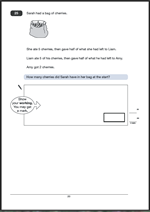Sarah had a bag of cherries…..Question 25 is one of those questions where you have to read it all carefully before starting and then proceed from the end, working backwards.

Suggested method:

The key to answering this question is to start at the end and work backwards.
Amy got 2 cherries.
Liam gave half of what he had left to Amy, so Liam must have had 4 left.
Liam had eaten 5 of his cherries so he must have had 5 + 4 = 9 cherries.
Sarah gave half of what she had left to Liam. This was 9 so she must have had 18 left.

Two marks for this question.

If the answer is incorrect one mark can be given for showing appropriate working out, such as
2 x 2 = 4          4 + 5 = 9          9 x 2 = wrong answer of 16              16 + 5 = wrong answer of 21.
The working out must be carried through to reach an answer.

This is very much at the level 5 end of the paper, so correct marks here could just make the difference between Level 4 and level 5.

Question 25 from SATs Paper A 2010

Question 25 answer and suggested method

## Year 2 investigation: 3 dice totals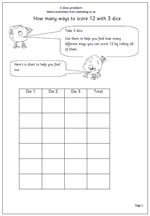Dice are a great resource to help with addition and logical thinking. This investigation looks at how many different ways a total of 12 can be made with three dice.

In fact this could be looked at in two different ways. If the dice were all different colours then there would be a great many more answers than if all three dice were the same and the order didn’t matter. For example the dice could be rolled as a 6, a 5 and a 1. With three similar dice  this would be the same as 1, 5 and 6 or 5, 6 and 1.

if the colours were different it could be recorded as

red: 6  blue: 5 and green 1

red 5, blue 6 green 1 etc

I would recommend with young children to keep to three similar dice as there are, in fact, very few answers. See the answer page for this.

Look for logical thinking and a well organised way of displaying results.

3 dice problem

## Complete multiplication number sentences (3)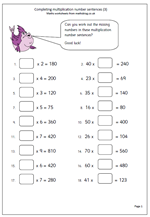This is the third worksheet in the mini series looking at using different strategies to work out missing numbers in multiplication number sentences. Again, it is worth pointing out that people who say they, ‘can’t do maths’, probably don’t have a wide range of mental strategies to tackle questions. All the questions on this worksheet look very similar but if you analyse how the brain works to answer them you will see what I mean.

Let’s look at a couple of examples:

1. Question: ? x 6 = 360

I instantly recognise the relationship between 6 and 36, knowing that 6 x 6 = 36. it then becomes very easy to multiply by 10 to reach the answer 60. This is all done in less than a second.

2. Question: 26 x ? = 104

This takes a little longer and at first glance I’m not certain. I then realised that 25 is a quarter of 100, so 25 x 4 is 100,therefore 26 x 4 would be 104.

In the first question I am making use of knowing the 6x table. In the second question I am rounding a number, working out the answer and adjusting.

Of course, there are other ways, probably just as good  and don’t forget to ask the children how they worked out the answers. You might be surprised!

Complete multiplication number sentences (3)

## KS2 Maths SAT questions 23 and 24 from 2010 Paper A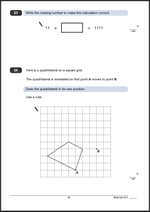To answer question 23 you need to find the missing number to make the calculation correct.

11 x ?? = 1111

One mark for this question.

Suggested method:
To make this easy a good understanding of the relationship between multiplication and division is needed. Simply divide 1111 by 11, which is probably best done using the short division method.

It is possible that some children will recognise the pattern of dividing by 11 and immediately know this answer.

Question 24 asks for a quadrilateral to be drawn in its new position after a translation.

One mark for this question.

Slight inaccuracies in drawing are allowed (within 2 mm radius of correct points).

Suggested method:

The key to this question is the word ‘translated’. A translation is a slide in a particular direction. There is no rotation or change in the shape size.

This shape has been slid 3 squares to the right and 3 squares up.

Using this knowledge mark each of the other three points and join them with a ruler.

Questions 23 and 24 from SATs Paper A 2010

Questions 23 and 24 answers and suggested methods

Why not visit ks2-maths-sats.co.uk for free SATs papers and a great SAT revision programme?

## More help with ordering decimals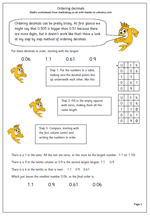I have published worksheets on ordering decimals before, but this page, lent to me from urbrainy.com is a very neat way of making sure that decimals are put in order correctly.

It suggests a very simple, step by step, approach which includes:

1. putting the numbers underneath each other, in a table, making sure that the decimals all line up.

2. filling any empty spaces with zeros, making them all the same length, with the same number of digits.

3. beginning to compare, starting with the units column.

Children who have not used decimals may well think that the size of a number depends on the number of digits so comparing decimals can be tricky, as a three digit number such as 0.19 is smaller than a 2-digit number such as 0.2.

Order decimals

## Year 6 maths worksheet: ordering decimalsOrdering decimals can be pretty tricky. At first glance we might say that 0.509 is bigger than 0.51 because there are more digits, but it doesn’t work like that! Have a look at my step by step method of ordering decimals.

Step 1: Put the numbers in a table, making sure the decimal points line up underneath each other.

Step 2: Fill in the empty squares with zeros, making them all the same length.

Step 3: Compare, starting with the first column (units) and writing the numbers in order.

This is quite a nifty way of making sure that you have got the numbers in order, although it is a little time consuming. Grids have been provided on the worksheets, but if a question like this comes up in the SAT tests it should be sufficient to just write the numbers in columns, not forgetting to put them in the answer page at the end!

Order decimals

## Year 4 mental arithmetic: multiplying by 4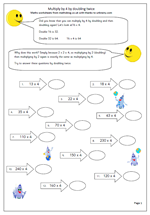Knowing the 4 times table is very important but using it is not always the most efficient way of finding an answer when using just mental methods. here we have a worksheet for year 4 children which looks at an alternative approach when multiplying by 4. This uses knowledge of the 2 times table to double and double again.

With some numbers this could be a better way. For example;

150 x4 can be done easily by doubling 150 to get 300 and then doubling 300 to get 600 – all done ‘in your head’ in less than a second!

Why not try this worksheet, to be found in the Year 4 calculating category.

Multiply by 4 by doubling

## KS2 Maths SAT question 22 from 2010 Paper AQuestion 22a and 22b look at interpreting pie charts.

For 22a  An acceptable answer is within the range of 13/100 to 1/5 inclusive.
This range includes the fractions 1/6 and 1/7.
Decimals can be accepted within the range 0.13 to 0.2 inclusive.
Percentages can be accepted within the range 13% to 20% inclusive.
One mark for this question.
Suggested method:
This can be thought of as a visual question. Split the circle in half by continuing the vertical line down (between Books and DVDs.).  From this it can be seen that DVDs are just about 1/3 of the half, so will be about 1/6 of the whole circle. Because it is only an estimate there is a fair range to be within.

For 22b Answers in the range of 500 to 800 inclusive.
One mark for this question.

Suggested method:
Again this can be done by comparing the size of the two. Book sales are about 3 times that of CDs. So if 200 CDs were sold then about 600 books were sold.

Question 22 from Maths Paper A 2010

Question 22 answers and suggested methods.

## Year 4 maths worksheet: multiplying by 5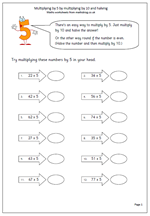By the end of year 4 children should be developing a wide range of strategies for working answers out ‘in their heads’. To be successful they do need plenty of practice with these strategies. One such technique is to be able to efficiently multiply 2-digit numbers by 5. Sometimes the best way to do this is to take the tens digit and multiply by 5 then do the same with the units. On other occasions it is easier to multiply the whole number by ten and then halve the answer; or, if the number is even, halve the number and multiply by ten.

This worksheet looks more closely at this .it is not always the best option, but it is ceertainly one that children should be confident with using.

Multiply by 5

## Year 3 maths worksheet: 4x table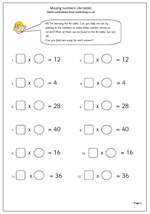This is a useful page for children who are beginning to get to grips with learning the 4x table. The questions are in pairs, with the same answer. This can reinforce the idea that multiplication can be done in any order; so 3 x 4 is the same as 4 x 3. There is only one question where this does not apply, and that is where 16 is the answer, which of course is the square of 4, or 4 x 4 . Another answer needs to be placed in the second number sentence, such as 2 x 8.

A good test of how well children know the 4x table will be the speed at which these worksheets are answered.

Thanks to urbrainy.com for letting me publish this resource and I can highly recommend their maths resources, which you can view on a free trial at www.urbrainy.com

Missing numbers 4x table